[MyHosting.com]   [KO4BB Home Page]   [Manuals Home Page]   [KO4BB Wiki]

# Differences

This shows you the differences between two versions of the page.

 — precision_timing:fractional_dividers_in_a_pld [2013/01/08 19:00] (current) Line 1: Line 1: + ====== Fractional Dividers in a PLD ====== + + //From the Time Nuts mailing list: // + + > A 10 MHz clock into a PIC gives a 400 ns/​instruction time. + > + > To produce 32.768 kHz you flip an output pin put every 38 + > instructions,​ except that 9632 times per second you make it 39 + > instructions instead. + > + + To figure out those sorts of ratios for static frequency dividers, Allan Herriman has written a nifty fractional divider script that generates VHDL/​Verilog code for CPLD/FPGA applications:​ + + http://​fractional-divider.tripod.com/​ + + It will generate dual modulus or phase accumulator based dividers, with various implementation options. + + To generate a 10 Mhz to 32.768 Khz with a frequency error tolerance of 1e-12 : + + fracn09.pl ​ -t 1e-12  10e6  32768 + + Which generates a file "​fracn.vhd":​ + <​code>​ + -- + -- Frequency Results (use_phase_accumulator = FALSE) : + --  Achieved Output Frequency: 32768 Hz. + --  Achieved Relative Frequency Error: 0 (0 ppm) + --  Achieved Frequency Error: 0 Hz. + -- + ​ + + <​code>​ + constant n              : positive := 305;  -- prescaler divides by n or n + 1 + constant a              : positive := 211;  -- this many counts of 305 + constant b              : natural ​ := 45;   -- this many counts of 306 + + ​ + + <​code>​ + -- + -- Design Parameters (use_phase_accumulator = FALSE) : + --  Approx 20 flip flops (9 in prescaler, 9 in controller and 2 retimes). + --  The recursive controller uses approx 17 flip flops. + --  The Dual-Modulus Prescaler uses ratios /305,/306 + --  The Output consists of 211 cycles of 305 input clocks, + --  and 45 cycles of 306 input clocks. + --  There are 256 output clocks for every 78125 input clocks. + -- +  ​ + + Brian +

precision_timing/fractional_dividers_in_a_pld.txt · Last modified: 2013/01/08 19:00 (external edit)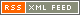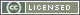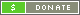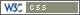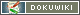##### Except as noted, this entire site Copyright © 2002-2017. KO4BB All rights reserved.